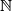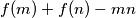### IMO Shortlist 2016 problem N6

Kvaliteta:
Avg: 0,0
Težina:
Avg: 8,0

Denote by$\mathbb{N}$ the set of all positive integers. Find all functions$f:\mathbb{N}\rightarrow \mathbb{N}$ such that for all positive integers$m$ and$n$, the integer$f(m)+f(n)-mn$ is nonzero and divides$mf(m)+nf(n)$.

Izvor: https://www.imo-official.org/problems/IMO2016SL.pdf